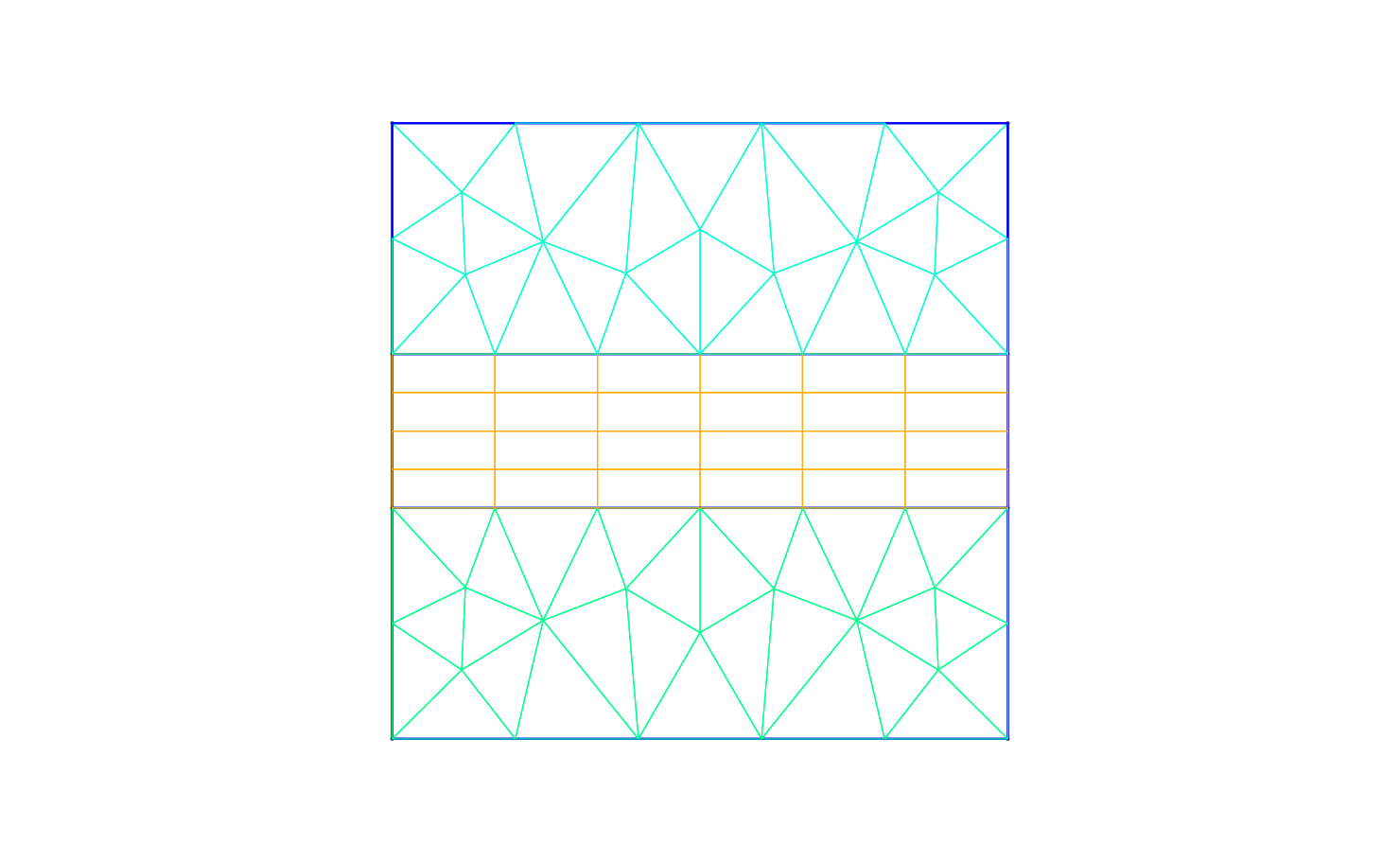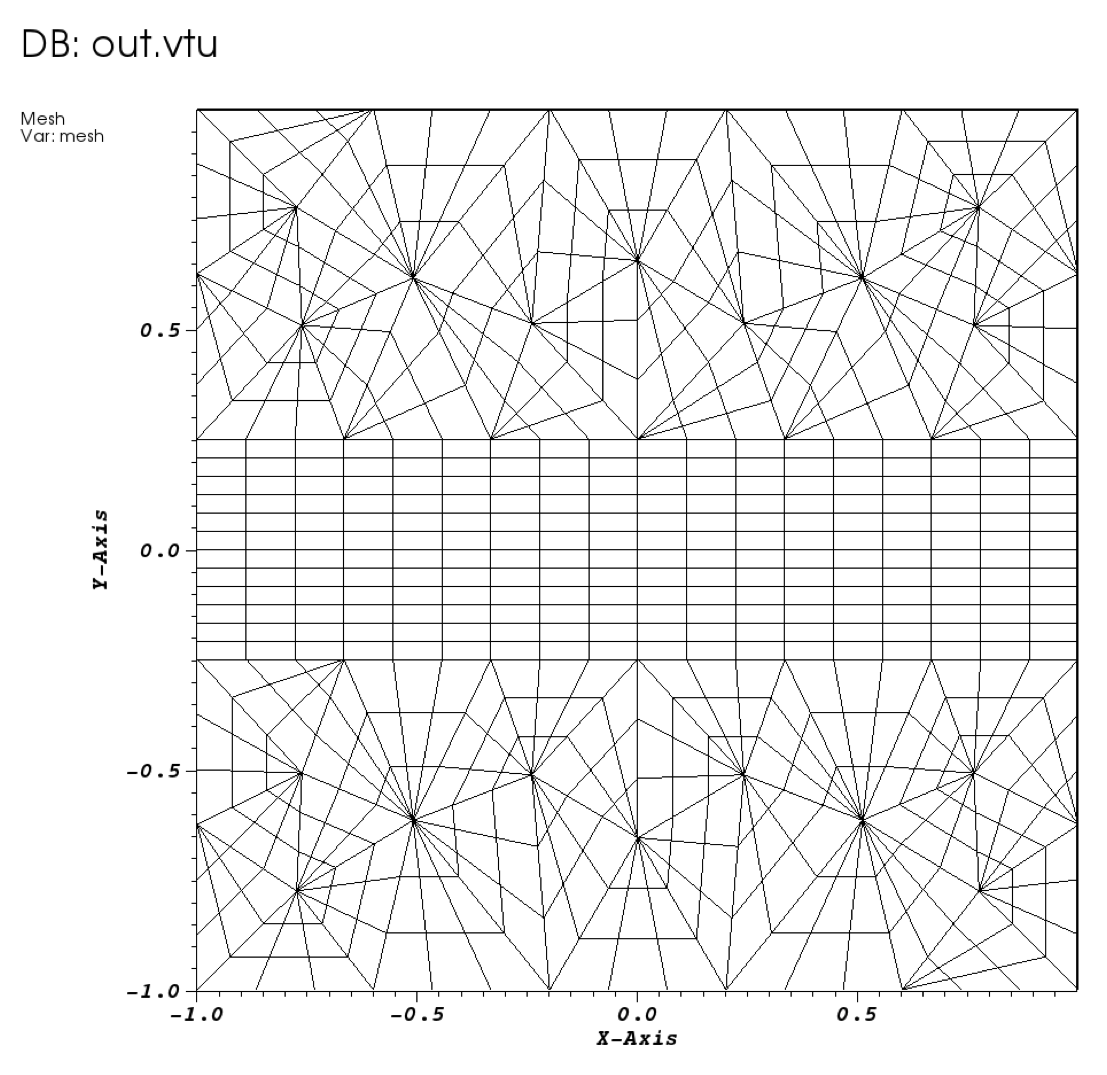## Chapter 2Pre-processing

As already mentioned to set up the probelm we have two step. The first is setting up a mesh in an input xml format consistent with Nektar++ as discussed in section 2.1. We also need to configure the problem initial, boundary and parameters which are discussed in 2.2.

### 2.1 Mesh generation

The first pre-processing step consists in generating the mesh in a Nektar++ compatible format. One option to do this is to use the open-source mesh-generator Gmsh to first create the geometry, that in our case is a square and successively the mesh. The mesh format provided by Gmsh shown in Fig. (2.1) - i.e. .msh extension - is not consistent with the Nektar++ solvers and, therefore, it needs to be converted.Figure 2.1: Mesh generated by Gmsh.

To do so, we need to run the pre-processing routine called `NekMesh` within Nektar++. This routine requires two line arguments, the mesh file generated by Gmsh, `ADR_mesh.msh`, and the name of the Nektar++-compatible mesh file that `NekMesh` will generate, for instance `ADR_mesh.xml`. The command line for this step is

Task: 2.1 Convert the .meh file into a Nektar++ input by calling

`\$NEK/NekMesh ADR_mesh.msh ADR_mesh.xml`

or

`\$NEK/NekMesh ADR_mesh.msh ADR_mesh.xml:xml:uncompress`

Note that by default the information is stored in Xml blocks of compressed data to reduce the size of large meshes. The second command above tells the converter `NekMesh` to write the file out in uncompressed format. The generated .xml mesh file is reported below and can also be found in the `completed` directory. It contains 5 tags encapsulated within the `GEOMETRY` tag, which describes the mesh. The first tag, `VERTEX`, contains the spatial coordinates of the vertices of the various elements of the mesh. The second tag, `EDGE` contains the lines connecting the vertices. The third tag, `ELEMENT`, defines the elements (note that in this case we have both triangular - e.g. `<T ID="0">` - as well as quadrilateral - e.g. `<Q ID="85">` - elements). The fourth tag, `COMPOSITE`, is constituted by the physical regions of the mesh called composite, where the composites formed by elements represent the solution sub-domains - i.e. the mesh sub-domains where we want to solve the linear advection problem (note that we will use these composites to define expansion bases on each sub-domain in section 2.2) - while the composites formed by edges are the boundaries of the domain where we need to apply suitable boundary conditions (note that we will use these composites to specify the boundary conditions in section 2.2). Finally, the fifth tag, `DOMAIN`, formally specifies the overall solution domain as the union of the three composites forming the three solution subdomains (note that the specification of three subdomain - i.e. composites - in this case is necessary since they are constituted by different element shapes). For additional details on the `GEOMETRY` tag refer to the User-Guide.

1<?xml version="1.0" encoding="utf-8" ?>
2<NEKTAR>
3   <GEOMETRY DIM="2" SPACE="2">
4      <VERTEX>
5        <V ID="0">2.00000000e-01 -1.00000000e+00 0.00000000e+00</V>
6        <V ID="1">5.09667784e-01 -6.15240515e-01 0.00000000e+00</V>
7        ...
8        <V ID="68">-1.00000000e+00 1.25000000e-01 0.00000000e+00</V>
9      </VERTEX>
10      <EDGE>
11        <E ID="0"> 0 1 </E>
12        <E ID="1"> 1 2 </E>
13        ...
14        <E ID="153"> 40 68 </E>
15      </EDGE>
16      <ELEMENT>
17        <T ID="0"> 0 1 2 </T>
18        <T ID="1"> 3 4 5 </T>
19        ...
20        <Q ID="85"> 146 93 153 151 </Q>
21     </ELEMENT>
22      <COMPOSITE>
23        <C ID="1"> T[0-30] </C>
24        <C ID="2"> Q[62-85] </C>
25        <C ID="3"> T[31-61] </C>
26        <C ID="100"> E[46,12,20,10,45] </C>
27        <C ID="200"> E[50,32,108,111,114,117,87,103] </C>
28        <C ID="300"> E[100,64,74,66,99] </C>
29        <C ID="400"> E[49,33,148,150,152-153,86,104] </C>
30      </COMPOSITE>
31      <DOMAIN> C[1,2,3] </DOMAIN>
32   </GEOMETRY>
33</NEKTAR>

After having generated the mesh file in a Nektar++-compatible format, `ADR_mesh.xml`, we can visualise the mesh. This step can be done by using the following Nektar++ built-in post-processing routine:

Task: 2.2 Convert the .xml file into a .vtu format by calling

`\$NEK/FieldConvert ADR_mesh.xml ADR_mesh.vtu`

Alternatively a tecplot .dat file can be created by changing the extension of the second file, i.e.

`\$NEK/FieldConvert ADR_mesh.xml ADR_mesh.dat`

This will produce a `ADR_mesh.vtu` file which can be directly read by the open-source visualisation tool called Paraview or VisIt. In Fig. 2.2 we show the mesh distribution for the mesh considered in this tutorial, `ADR_mesh.xml`.Figure 2.2: Mesh distribution with local polynomial subdivision

Before configuring the input files, if we want to use periodic boundary conditions, we need to make sure that the edges of the two periodic boundaries (i.e. xb = [-1,1],yb) are properly aligned. Gmsh and the `NekMesh` routine within Nektar++ does not guarantee proper alignment. However, `NekMesh` provides a module, called `peralign`, that enforces the reordering of pair of edges (for more details refer to the User-Guide. We can apply this by using the following command:

Task: 2.3 Convert the .xml file into a .xml file with periodic edges aligned

```\$NEK/NekMesh -m peralign:surf1=200:surf2=400:dir=x ADR_mesh.xml ADR_mesh_aligned.xml```

where `-m peralign` is selecting the module for aligning the edges which are specified by `surf1` and `surf2` (their IDs in this case are 200 and 400) and `dir` is the direction to which the two periodic edges are perpendicular (in this case x). Note that since we have not used the extension `:xml:uncompress` the blocks of data in this file are now stored in compressed format.

After having typed the last command, we have a mesh, `ADR_mesh_aligned.xml`, which is fully compatible with Nektar++ and which allows us applying periodic boundary conditions without encountering errors.

We can therefore now configure the conditions: initial conditions, boundary conditions, parameters and solver settings.

### 2.2 Configuring problem definitions

To set the various problem parameters, the solver settings, initial and boundary conditions and the expansion basese, we use the file called `ADR_conditions.xml`, which can be found within the `tutorial` directory for this tutorial. This new file contains the `CONDITIONS` tag where we can specify the parameters of the simulations, the solver settings, the initial conditions, the boundary conditions and the exact solution and contains the `EXPANSIONS` tag where we can specify the polynomial order to be used inside each element of the mesh, the type of expansion bases and the type of points.

We begin to describe the `ADR_conditions.xml` file from the `CONDITIONS` tag, and in particular from the boundary conditions, initial conditions and exact solution sections:

1<CONDITIONS>
2   ...
3   ...
4   ...
5   <VARIABLES>
6      <V ID="0"> u </V>
7   </VARIABLES>
8
9   <BOUNDARYREGIONS>
10      <B ID="0"> C </B>
11      <B ID="1"> C </B>
12      <B ID="2"> C </B>
13      <B ID="3"> C </B>
14   </BOUNDARYREGIONS>
15
16   <BOUNDARYCONDITIONS>
17      <REGION REF="0">
18        <D VAR="u" USERDEFINEDTYPE="TimeDependent"
20      </REGION>
21      <REGION REF="1">
22        <P VAR="u" VALUE="" />
23      </REGION>
24      <REGION REF="2">
25         <D VAR="u" USERDEFINEDTYPE="TimeDependent"
27      </REGION>
28      <REGION REF="3">
29        <P VAR="u" VALUE="" />
30      </REGION>
31   </BOUNDARYCONDITIONS>
32
33   <FUNCTION NAME="InitialConditions">
34      <E VAR="u" VALUE="sin(k*x)*cos(k*y)" />
35   </FUNCTION>
36
40   </FUNCTION>
41
42   <FUNCTION NAME="ExactSolution">
44   </FUNCTION>
45</CONDITIONS>

In the above piece of `.xml`, we first need to specify the non-optional tag called `VARIABLES` that sets the solution variable (in this case u).

The second tag that needs to be specified is `BOUNDARYREGIONS` through which the user can specify the regions where to apply the boundary conditions. For instance, ```<B ID="0"> C </B>``` indicates that composite 100 (which has been introduced in section 2.1) has a boundary ID equal to 0. This boundary ID is successively used to prescribe the boundary conditions.

The third tag is `BOUNDARYCONDITIONS` by which the boundary conditions are actually specified for each boundary ID specified in the `BOUNDARYREGIONS` tag. The syntax `<D VAR="u"` corresponds to a `D`irichlet boundary condition for the variable `u` (note that in this case we used the additional tag `USERDEFINEDTYPE="TimeDependent"` which is a special option when using time-dependent boundary conditions), while `<P VAR="u"` corresponds to `P`eriodic boundary conditions. For additional details on the various options possible in terms of boundary conditions refer to the User-Guide.

Finally, `<FUNCTION NAME="InitialConditions">` allows the specification of the initial conditions, `<FUNCTION NAME="AdvectionVelocity">` specifies the advection velocities in both the x- and y-direction (for this two-dimensional case) and is a non-optional parameters for the unsteady advection equation and `<FUNCTION NAME="ExactSolution">` permits us to provide the exact solution, against which the L2 and L errors are computed.

After having configured the `VARIABLES` tag, the initial and boundary conditions, the advection velocity and the exact solution we can complete the tag `CONDITIONS` prescribing the parameters necessary (`PARAMETERS`)and the solver settings (`SOLVERINFO`):

1<CONDITIONS>
2   <PARAMETERS>
3      <P> FinTime = 1.0 </P>
4      <P> TimeStep = 0.001 </P>
5      <P> NumSteps = FinTime/TimeStep </P>
6      <P> IO_CheckSteps = 100 </P>
7      <P> IO_InfoSteps = 100 </P>
8      <P> advx = 2.0 </P>
9      <P> advy = 0.0 </P>
10      <P> k = 2*PI </P>
11   </PARAMETERS>
12
13   <SOLVERINFO>
15      <I PROPERTY="Projection" VALUE="DisContinuous" />
17      <I PROPERTY="UpwindType" VALUE="Upwind" />
18      <I PROPERTY="TimeIntegrationMethod" VALUE="ClassicalRungeKutta4"/>
19   </SOLVERINFO>
20   ...
21   ...
22   ...

In the `PARAMETERS` tag, `FinTime` is the final physical time of the simulation, `TimeStep` is the time-step, `NumSteps` is the number of steps, `IO_CheckSteps` is the step-interval when a output file is written, `IO_InfoSteps` is the step-interval when some information about the simulation are printed to the screen, `advx` and `advy` are the advection velocities Vx and Vy, respectively and `k` is the κ parameter. Note that `advx`, `advy` and `k` are used in the boundary and initial conditions tags as well as in the specification of the advection velocities.

In the `SOLVERINFO` tag, `EQTYPE` is the type of equation to be solved, `Projection` is the spatial projection operator to be used (which in this case is specified to be ‘DisContinuous’), `AdvectionType` is the advection operator to be adopted (where the `VALUE` ‘WeakDG’ implies the use of a weak Discontinuous Galerkin technique), `UpwindType` is the numerical flux to be used at the element interfaces when a discontinuous projection is used, `TimeIntegrationMethod` allows selecting the time-integration scheme. For additional solver-setting options refer to the User-Guide.

Finally, we need to specify the expansion bases we want to use in each of the three composites or sub-domains (`COMPOSITE=".."`) introduced in section 2.1:

1<EXPANSIONS>
2   <E COMPOSITE="C" NUMMODES="5" TYPE="MODIFIED" FIELDS="u" />
3   <E COMPOSITE="C" NUMMODES="5" TYPE="MODIFIED" FIELDS="u" />
4   <E COMPOSITE="C" NUMMODES="5" TYPE="MODIFIED" FIELDS="u" />
5</EXPANSIONS>

In particular, for all the composites, `COMPOSITE="C[i]"` with i=1,2,3 we select identical basis functions and polynomial order, where `NUMMODES` is the number of coefficients we want to use for the basis functions (that is commonly equal to P+1 where P is the polynomial order of the basis functions), `TYPE` allows selecting the basis functions `FIELDS` is the solution variable of our problem and `COMPOSITE` are the mesh regions created by Gmsh. For additional details on the `EXPANSIONS` tag refer to the User-Guide.

Task: 2.4 Generate the file `ADR_conditions.xml` or copy it from the `completed` directory.